# Chapter 17 - While Loops¶

## 1.1. Beat until smooth¶

In the last two chapters we have been learning about for-loops. A for-loop is the simplest solution when you start out with a fixed sequence, and you want to perform some action once for each item.

for each cupcake
put on frosting and sprinkles


You always know the number of iterations in advance, or at least the maximum number of iterations, since you could exit the loop early.

But there are situations when you don’t start out with a known sequence of items to act upon, and you don’t know in advance how many times something is going to happen. For example, the directions for making the cupcakes might have said something like

add two eggs and mix until smooth


So, how much are we supposed to mix? As long as it is still lumpy, we have to mix it some more. You have probably experienced similar situations.

drive around the block until you have found a parking space
keep practicing until you can play the piece without mistakes


We can describe activities like this a bit more precisely using the word “while”.In programming, situations like this call for a more general type of loop, called a while-loop. In Python, a while-loop is written with the while keyword: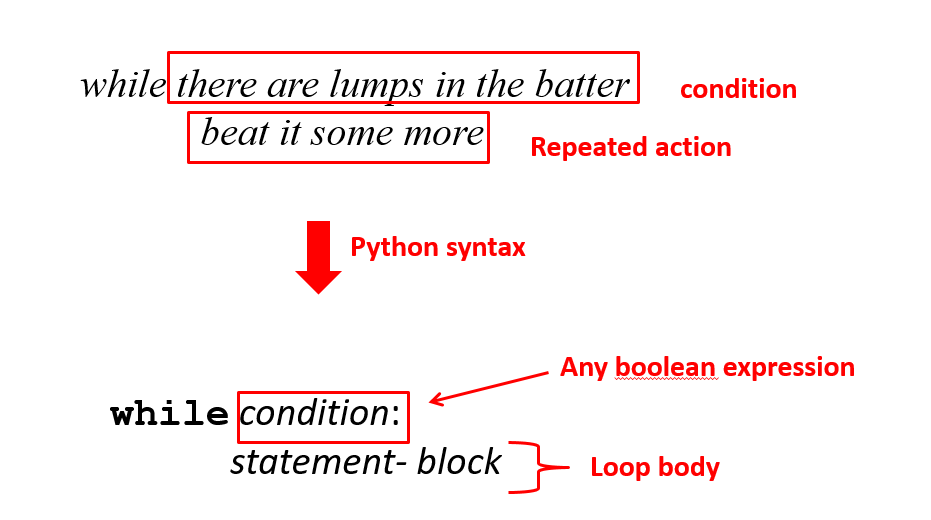As with a for-loop, the statement block is known as the loop body. The way it works is really simple. If the condition is true, the loop body gets executed. After the loop body executes, we go back and re-check the condition. If the condition is still true, the loop body is executed again. And so on. And so on. And so on.

As a simple example to start with, let’s write a loop that prints the characters in a string one at a time. We did this already using a for-loop. It was easy. We basically just said, “For each character in the string, print it.”

To do the same thing with a while-loop, we have to work a little bit harder. We have to keep track of the index for the next character, and increment the index ourselves.

To make sure we understand what’s going on here, let’s trace through this code using a table as we did in the last unit. Before entering the loop, the initial value of index is zero, and the variable ch is undefined.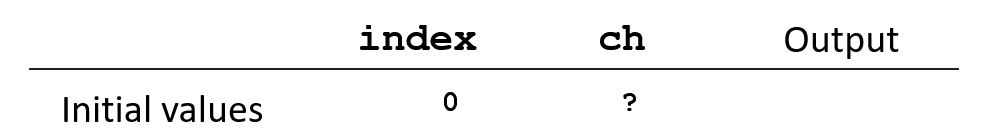Now, we check the condition. The length of “Steve” is 5. Is index less than 5? The answer is yes, so we enter the loop body. ch gets the character at index 0, which is “S”, and then index is incremented by 1. We write down the values of the variables at the end of the loop body, and we’ll also show the output in the table.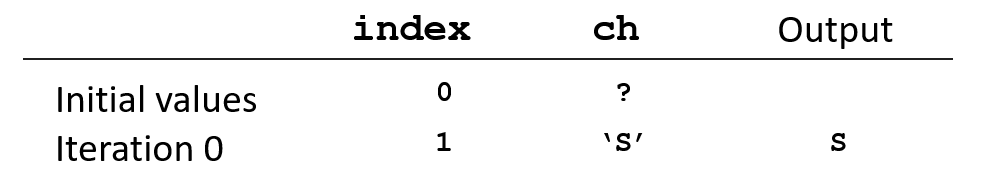Now, we go back and check the condition again. index is 1. Is 1 less than 5? Yes, so we enter the loop body. ch gets the character at index 1, which is “t”, and then index is incremented by 1.

Check the condition again. Index is still less than 5, so we execute the loop body again.

Check the condition, and execute the loop body again.

And again!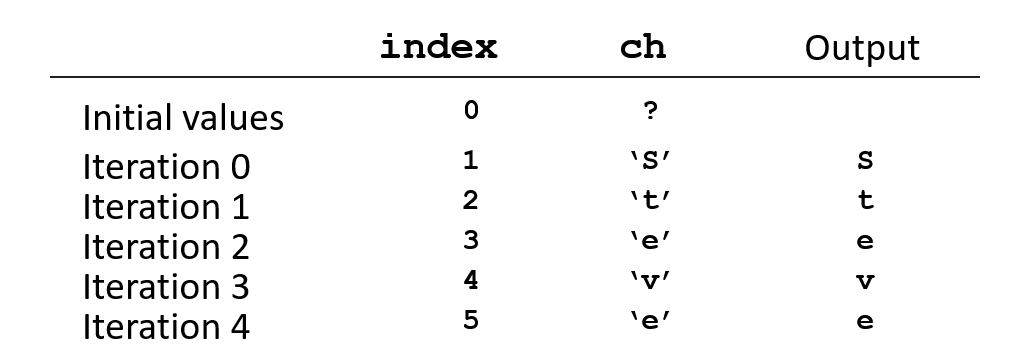Now we go back and check the condition again with index equal to 5. Since 5 is not less than 5, the condition is false and we don’t enter the loop body, so the loop is finished.

Here’s an important thing to notice. What would happen if we forgot to increment the index in the loop body?

index = 0
word = "Steve"
while index < len(word):
ch = word[index]
print (ch)


If index stays at zero, our table would start out looking like this: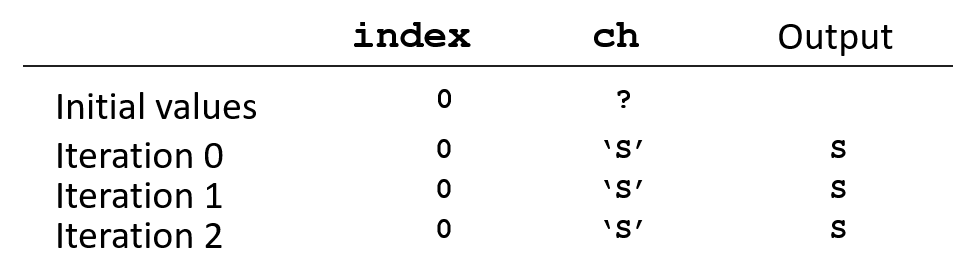The condition “index is less than the length of ‘Steve’” is always true, so the loop body keeps executing over and over again, printing the character “S”. Forever! This is called an infinite loop.

Sometimes we write infinite loops on purpose, for applications that are supposed to keep running all the time, like web servers and telephone switches. But sometimes we get infinite loops from programming errors. One thing you should be sure to know is: how do you stop an infinite loop? In most environments, the key combination “Control-C” will work.

NOTE: Your browser will most likely crash if you try this in an activecode window. Similarly, if you end up with an infinite loop in Wing 101, you’ll have to forcefully shut down the application. (In Windows, use Ctrl-Alt-Delete to bring up the Task Manager. In OS X you can right-click on Wing in the dock and select Force Quit.)

It’s also possible for the opposite to happen. What if we had this, where the inequality is accidentally reversed.

The condition starts out false, so the loop body will never execute, not even once.

You can see that while-loops are a bit more difficult to write than for-loops. So why would we ever want to use them? We need while-loops for situations in which we don’t have a fixed list of items to iterate over or we don’t know the number of iterations in advance.

## 1.2. Examples of while-loops¶

Remember that in the last chapter, we wrote a for-loop to find the sum of a sequence of numbers. What if instead of having a known sequence, the numbers were being entered one at a time from the keyboard? We don’t necessarily know in advance how many values there will be. Instead, let’s assume that the user can enter a “q” to indicate that there are no more values to enter. We’ll just print the total when the user is done. Logically we want something like this: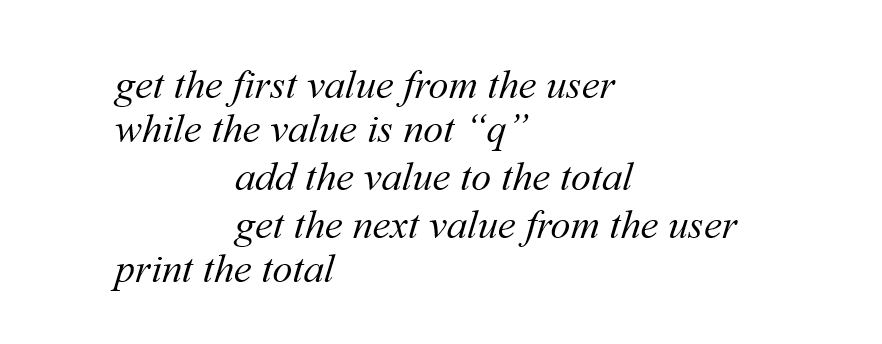Maybe we want the average instead, so we have to divide by the number of entries. Hm, we don’t actually know the number of entries. We can’t just use the len function on a list, because we don’t have a list! We’ll have to count the values as they are entered. We just initialize a counter to zero before the loop, and add 1 each time we add something to the total.

Here is another kind of example in which we don’t know the number of iterations in advance. In the last chapter, we wrote a function that checks whether a given number is prime. Large prime numbers are extremely useful, for example, they are used extensively in computer security. Suppose we want to write a function that helps us find large prime numbers. Given any number n, we want to return the next prime number that is larger than n. We’ll try n + 1, n + 2, n + 3, and keep going until we find a number that is prime. How many numbers will we have to try before we find a prime? There is no way to predict in advance, so we have to use a while-loop. Our logic might look like this:

start with p = n + 1
while p is not prime
increment p by 1


The loop will execute over and over again until the condition “p is not prime” is false, meaning that p really is prime. So when the loop exits, we can return the number p.

## 1.3. Designing a while-loop¶

There is no doubt that writing while-loops is one of the most difficult aspects of programming. If you think about the examples we’ve done, you’ll notice that all while-loops seem to have three things in common.

• There is a loop body which may be repeated
• There is some initialization before the loop starts
• There is a condition that determines whether to execute the loop body again

These three pieces are the answers to three questions: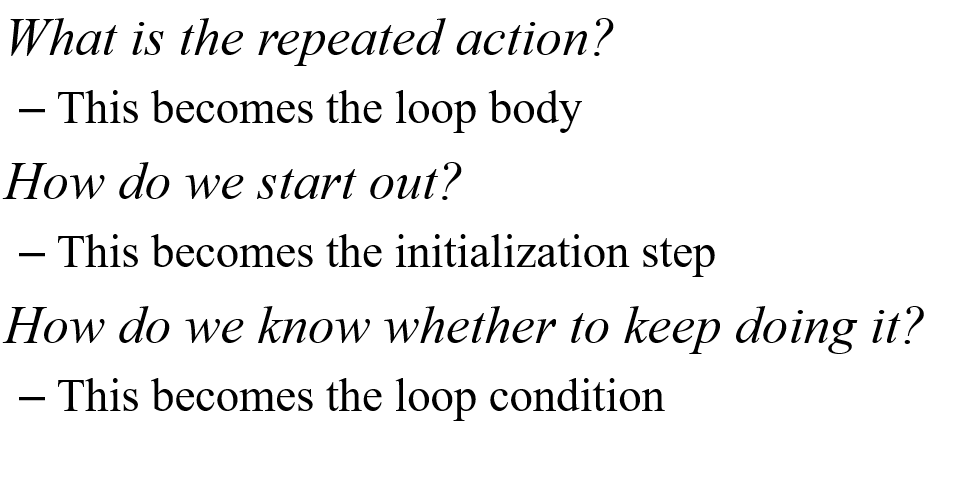When writing a while-loop you can use these three questions as a guide.

## 1.4. Example: an interactive game¶

To illustrate how we might think about loops this way, let’s write a simple interactive guessing game. While-loops come up a lot in interactive programming because we usually can’t predict what a user is going to do. Our script will pick a random number between 1 and 100, and the user will try to guess it. Whenever she guesses wrong, will give a hint as to whether it was too high or too low. We might envision a sample interaction like this: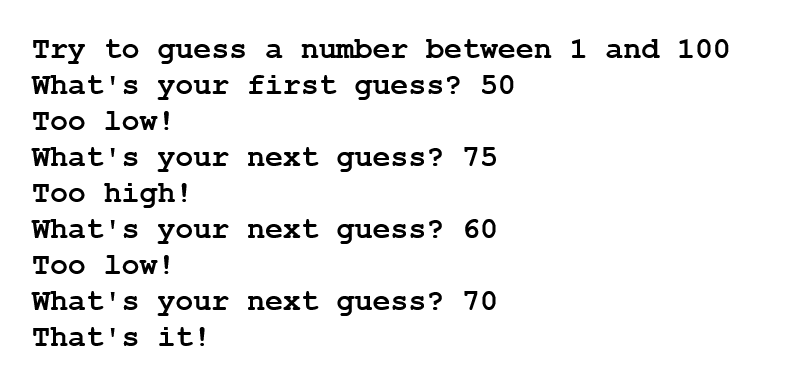Let’s think about the loop for the guessing game by asking the three questions.

Step 1: What is the repeated action?

Check whether the guess is too low or too high, and print a message. Then, get the user’s next guess.

Step 2: How do we start out?

We need to generate the secret number, and we need to get the user’s first guess.

Step 3: How do we know whether to keep doing it?

The user’s guess isn’t the secret number.

For step 1, if we define a variable secret to represent the secret number, and a variable guess to represent the user’s guess, the code for the repeated action could be written like this. The repeated action will be the body of a while-loop. We’ll write the loop body, and worry about the condition later.

while ________:
if guess < secret:
print("Too low!")
else:
print("Too high!")
guess = int(input("What's your next guess?"))


For step 2, we can use the randrange() function to initialize the secret number, and then read the user’s first guess.

secret = random.randrange(1, 101)
print("Try to guess a number between 1 and 100")
guess = int(input("What's your first guess?"))


For step 3, we just need a condition that says that guess isn’t equal to secret:

while guess != secret:


We’ll put the code into a function play_game. We need one additional line after the loop, to tell the user when she is successful. Try this code in Wing 101(the repeated inputs don’t work well in an activecode window).

import random

# Plays one round of a number-guessing game
def play_game():
secret = random.randrange(1, 101)
print ("Try to guess a number between 1 and 100")
guess = int(input("What's your first guess? "))
while guess != secret:
if guess < secret:
print ("Too low!")
else:
print ("Too high!")
guess = int(input("What's your next guess? "))
print ("That's it!")

# Call the function
play_game()


We might add a user interface for this function so that the user can choose whether to play again, or quit. One way to do this is to provide a menu of choices, which might look like this.

We can generate the menu with a simple function:

Since we can’t predict in advance how many times the user wants to play the game, we need a while loop. We’ll write the loop using asking the usual three questions:

Step 1: What is the repeated action?

Perform the user’s choice, display the menu, and allow the user to select an option.

Step 2: How do we start out?

Display the menu and allow the user to select an option.

Step 3: How do we know whether to keep doing it?

We keep doing it as long as the user doesn’t enter a “q”.

For step 1, we have to check what the user entered and perform the operation. Since there is only one choice besides “quit”, we are really just checking whether the entry is valid. If the user really does enter a “p”, we call the play_game function to play one round. The repeated actions become the body of the loop.

while ________:
if entry == "p":
play_game()
else:
entry = input("Enter your choice: ")


For step 2, we need to display the menu and get the user’s first selection.

print("Welcome to the guessing game")
entry = input("Enter your choice: ")


For step 3, the loop condition simply checks that the entry is not a “q”.

while entry != "q":


When the pieces are put together the whole script looks like this. Copy and paste into Wing 101 to try it (the repeated input statements don’t work well in activecode).

import random

# Plays one round of a number-guessing game
def play_game():
secret = random.randrange(1, 101)
print ("Try to guess a number between 1 and 100")
guess = int(input("What's your first guess? "))
while guess != secret:
if guess < secret:
print ("Too low!")
else:
print ("Too high!")
guess = int(input("What's your next guess? "))
print ("That's it!")

# Prints a menu for the user interface
print ("  p) Play the guessing game")
print ("  q) Quit")

# Main loop for a number-guessing game
def run_guessing_game():
print ("Welcome to the guessing game")
entry = input("Enter your choice: ")

while entry != "q":
if entry == "p":
play_game()
else:
print ("Please select p or q")